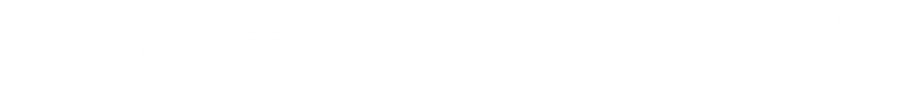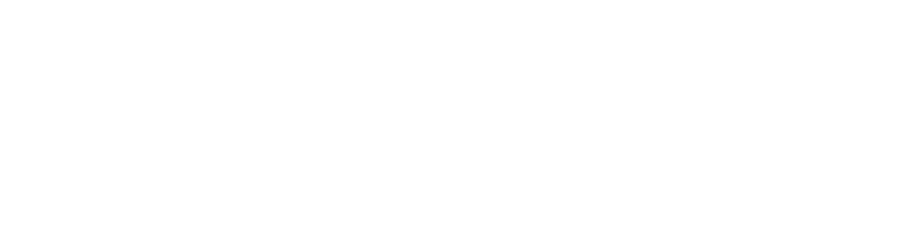# Heroic Triangles

How do you calculate the area of a triangle? You probably know the formula of “half the base multiplied by the vertical height”.This formula works well when you have information about the base and the perpendicular height, but sometimes the triangles you encounter are scalene, and with inconvenient rotations. You can get around this issue by reverting to trigonometry and calculating angles, but this can sometimes be a little burdensome.There is a formula for calculating the area of a triangle, using just the lengths of the sides. This formula is given the name Heron’s Formula, or sometimes the Hero Formula, after it’s first documented discoverer*, the Hero of Alexandria, a prolific mathematician and engineer (c. 10 AD – 70AD).
*There is some speculation that the formula was known by Archimedes a couple of centuries earlier.
Heron's formula states that the area of a triangle whose sides have lengths a, b, and c is :(s is often called the semi-perimeter). Interestingly, I don't remember ever having been taught this equation in school!

## ProofThe derivation of this formula is pretty easy using Pythagoras. Dropping a perpendicular we have two equations:Combining these we get:Using difference of two squares:We know the area of the triangle is ½ × h × c, giving the result:## Try it out

Drag the vertices around to make triangles. If an edge is integer, the label at the top turns green. If you create a Heroic Triangle, the triangle turns green.

## Heroic Triangles

We're all familiar with Pythagorean triplets. These are right-angle triangles that have all integer sides. The smallest is the {3,4,5} triangle. Heroic (Heronian) triangles are triangles that have integer length sides and an integer area.
All Pythagorean triplets are Heronian triangles (this has to be the case as the area is half the product of the two shorter sides; at least one of which must be even).
Heronian triangles don't have to be right angle. They can be isoceles, such as {5,5,6}, which can be formed by joining two Pythagorean {3,4,5} triangles back-to-back along the edge of length 4, or {5,5,8} by joining along the edge of length 3.
They can also be scalene, such as {5,29,30}, which can't be made from gluing together two Pythagorean triangles. These are given the name Indecomposable Heronian Triangles.
It's not possible for Heronian Triangle to be equilateral, but some triangles get very close, such as {51,52,53}, {2701,2702,2703}, {198924689265123,198924689265124,198924689265125}.
The total perimeter of a Heronian triangle is always an even number, and the semiperimeter can never be prime. Their areas are also always divisible by six. Every angle in a Heronian triangle has a rational sin, which you can see from the sine area formula.
There's a great Numberphile video about 'Superhero' triangles, which are the (limited number of) Heronian triangles that have an area that is also equal to their perimeter.